Rounded objects - Profiles

The function that defines the field has a profile parameter, p:

R-Function(p,Surface definition).

This takes a value between -1 and 1. The integer values (actually <= -1 and >= 1) are special cases which are illustrated by the following examples: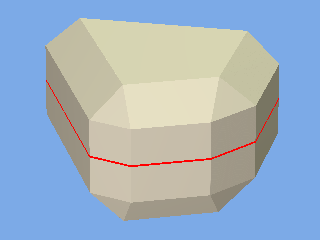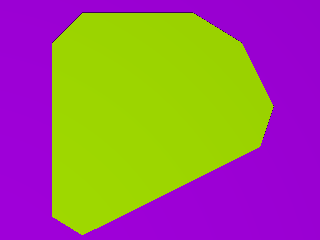p = -1 This gives a bevel with sharp corners, ie no rounding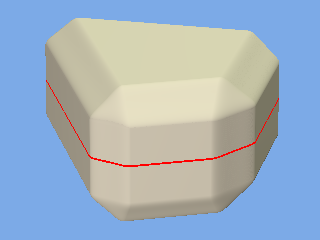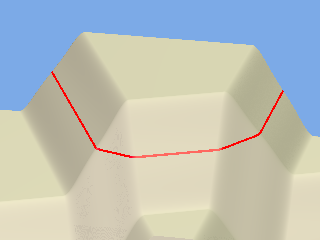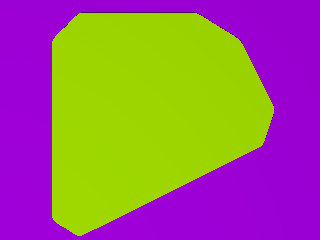p = -0.9 This is nearly flat with rounded corners. Less than this corner gets a bit concave, probably not useful. As p is increased the corner gets less flat until...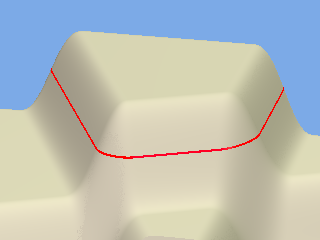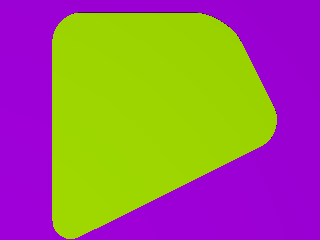p = 0.6 Here the corner is very close to round. Beyond this it gets a little bit sharper but probably not very useful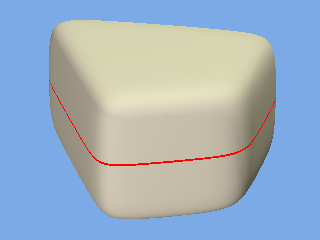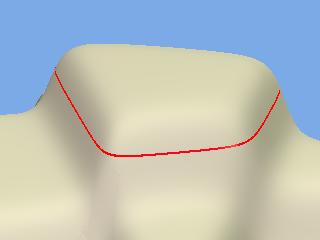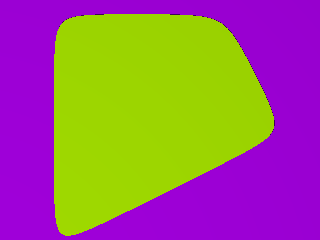p = 1 The corner is hyperbolic. It follows a curve which is assymptotic to the surfaces. This means that there are no flat surfaces anywhere.

The orange line in the left hand figure shows where a cross-section has been taken. The middle figure shows the field strength at this cross section and the right hand figure is the cross-section.

Note that the radius of the corner varies with the angle at which the planes meet. It is equal to the parameter r when the surfaces are at right angles. (This is different to rounds in CAD where they are exactly round and have the defined radius regardless of angles of the surfaces.)

The PovRay code used to produce these figures is:

// or send a letter to Creative Commons, 444 Castro Street, Suite 900, Mountain View,
// California, 94041, USA.

// Rounded objects: Illustration of the range of corner profiles

// Vers: 1.00
// Date: 17 Oct 2016
// Auth: John Greenwood

#version 3.7 ;
global_settings {assumed_gamma 1.0 }
#include "Rounded_Objects.inc"
#macro View_Setup(Number)
#if (Number=1)
camera {location <-30,20,-40> angle 5 look_at <0,0,0> }
light_source {<-5,20,-20> colour rgb<1,1,1>}
#elseif(Number=2)
camera {location <50,20,12> angle 7 look_at <0,0,.3> }
light_source {<30,5,-0> colour rgb<.9,.9,1>}
light_source {<-10,45,10> colour rgb<.9,1,.9>}
#else
camera {location <-40,20,40> angle 2 look_at <1,-1,0> }
light_source {<50,50,10> colour rgb<1,1,1>}
#end
background { color rgb<0.2, 0.4, 0.8> }
#end

//#declare Mode = "Section";
//#declare Mode = "Field";
#declare Mode = "ThreeD";
#declare View_Spec = 2;

//------------------------------------------------------------------------------------------

#declare R_Object1 =
function(p,r,x,y,z){
+R_function(p,((2*x+z)/sqrt(4+1) -2.5) /+r)
+R_function(p,((x-2*z)/sqrt(4+1) -1.6 ) /+r)
+R_function(p,(x +2.5) /-r)
+R_function(p,(z -2.5 ) /+r)
+R_function(p,(y +1.5) /-r)
+R_function(p,(y -1.5) /+r)

}

#declare Profile = -1; // change this to change the shape of the corner. 0.6 gives very close to circular
#declare Radius = .7; // radius when the corner is circular and surfaces are perpendicular

#if(Mode = "ThreeD")
View_Setup(View_Spec)

isosurface {
function {
// start of rounded object ****************************************

// end of rounded object **************************

}
threshold 1
contained_by { box {<-4.2,-3.2, -4.2>, <4.2,3.2,4.2>} }
texture {pigment {color rgb < 1, 0.9, 0.65>}}

}

Paint_Threshold()
#else
#if (Mode = "Section")
camera {location <0,99,0> angle 4.3 look_at <0,0,0> }
light_source {<-5,20,-5> color rgb<1,1,1>}
#else
View_Setup(View_Spec)
#end

isosurface {
function {
// start of rounded object. This the same as above except that y has been replaced by 0 ****************************************

// end of rounded object definition **************************

#if(Mode = "Section")
}
threshold 1
contained_by { box { <-3.2,.02,-3.2>,<3.2,0,3.2>} }
texture {pigment {color rgb < .5, 1, 0>}}

}

box{<-4,-1,-4>,<4,0,4> pigment{color rgb<.5,0,1> }}

#else
+y
} // the Y axis represents field strength and the orange line shows the threshold
threshold 1